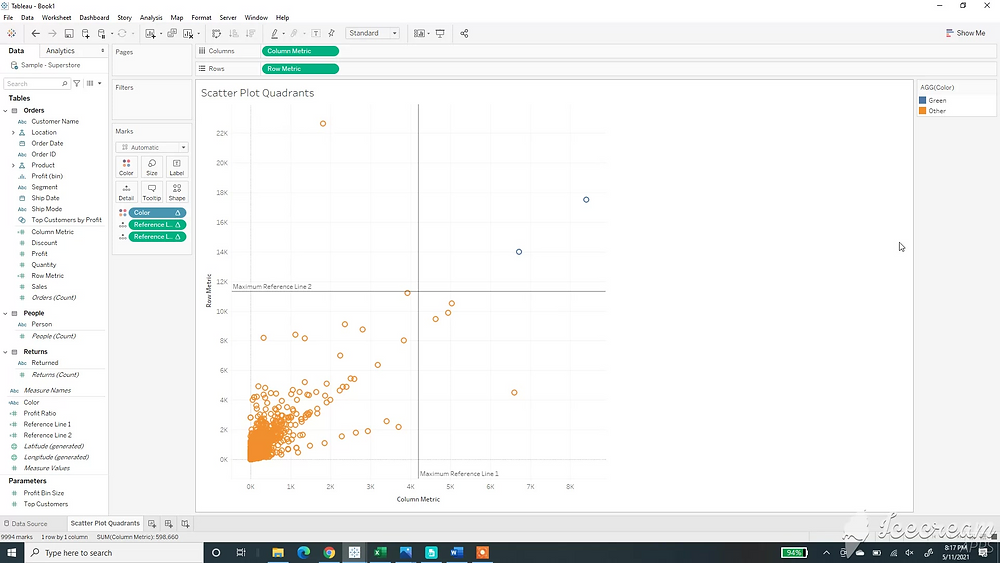Search
•Robert Breen## Step 1:

Build a scatter Plot using a metric on columns and a metric on rows. Unselect aggregated measures to finalize the scatter plot.## Step 2:

Create two calculations for reference lines.

window_max(SUM([Column Metric]))/2

window_max(SUM([Row Metric]))/2## Step 3:

Add the reference line metrics as a reference line on the x axis and the y axis. This will give you a quadrant like view.## Step 4:

Use this calculation to color the marks in the quadrant. Continue with elseif’s if you want to color other quadrants within the scatter plot.

if SUM([Column Metric])>= [Reference Line 1] and SUM([Row Metric]) >= [Reference Line 2] then "Green" else "Other" END54 views1 comment

See All